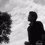# Proposal to RMM

Let $\Re(k)>0$ and if $\displaystyle\sum_{1\leq n\leq m \leq \infty} \frac{1}{n^2m+m^2n+kmn} =\frac{(H_{k+1})^2-\psi^1(k+\alpha)}{k+\beta}+\frac{\pi^{\alpha}}{\lambda(k+\beta)} \textbf{and}$ $\displaystyle\sum_{n=0}^{\infty}\sum_{q=0}^n \frac{x^n}{(q+b)\sqrt{q+b+1}+(q+b+1)\sqrt{q+b}}=\frac{1}{\sqrt{b}(1-x)}-\Phi\left(x,\frac{1}{\alpha},b+1\right)$ where $b\in\mathbf{N}$ and $|x|<1$ then prove that $\Phi\left(\beta-\alpha , \beta,(\alpha+2\beta +\lambda)^{-1} \right)$$= \frac{2\pi}{\sqrt5-1}+\sqrt{\phi+2}\log\left(\theta-\frac{8\theta}{4+\sqrt{10-2\sqrt5} +\sqrt{15}+\sqrt{3}}\right)+2^{-1}\sqrt{10-2\sqrt5 }\log\left(\frac{\sqrt 3\theta -1}{\theta +\sqrt 3}\right)$ and $\theta =\sqrt{\frac{8+\sqrt{10-2\sqrt 5}+\sqrt {15}+\sqrt{3}}{8-\sqrt{10-2\sqrt{5}}-\sqrt{15}-\sqrt{3}}}=\frac{8+\sqrt{10-2\sqrt 5}+\sqrt{15}+\sqrt{3}}{36-4\sqrt{5}-4\sqrt{6(5+\sqrt 5)}}$ where $\Phi(z,s,a)$ is Lerch Transcendent function , $H_k$ is the Kth Harmonic number, $\psi^1(x)$ is the trigamma function and $\phi$ is the Golden ratio.

This is one of my proposed problem to Romanian Mathematical Magazine.Note by Naren Bhandari
1 year, 3 months ago

This discussion board is a place to discuss our Daily Challenges and the math and science related to those challenges. Explanations are more than just a solution — they should explain the steps and thinking strategies that you used to obtain the solution. Comments should further the discussion of math and science.

When posting on Brilliant:

• Use the emojis to react to an explanation, whether you're congratulating a job well done , or just really confused .
• Ask specific questions about the challenge or the steps in somebody's explanation. Well-posed questions can add a lot to the discussion, but posting "I don't understand!" doesn't help anyone.
• Try to contribute something new to the discussion, whether it is an extension, generalization or other idea related to the challenge.
• Stay on topic — we're all here to learn more about math and science, not to hear about your favorite get-rich-quick scheme or current world events.

MarkdownAppears as
*italics* or _italics_ italics
**bold** or __bold__ bold
- bulleted- list
• bulleted
• list
1. numbered2. list
1. numbered
2. list
Note: you must add a full line of space before and after lists for them to show up correctly
paragraph 1paragraph 2

paragraph 1

paragraph 2

[example link](https://brilliant.org)example link
> This is a quote
This is a quote
    # I indented these lines
# 4 spaces, and now they show
# up as a code block.

print "hello world"
# I indented these lines
# 4 spaces, and now they show
# up as a code block.

print "hello world"
MathAppears as
Remember to wrap math in $$ ... $$ or $ ... $ to ensure proper formatting.
2 \times 3 $2 \times 3$
2^{34} $2^{34}$
a_{i-1} $a_{i-1}$
\frac{2}{3} $\frac{2}{3}$
\sqrt{2} $\sqrt{2}$
\sum_{i=1}^3 $\sum_{i=1}^3$
\sin \theta $\sin \theta$
\boxed{123} $\boxed{123}$

## Comments

Sort by:

Top Newest

I am in class 10 and I do not know about any of the operators mentioned in question. But it seems interesting! ;)

- 1 year, 3 months ago

Log in to reply

I started brilliant.org by the end of 2016 and now I think I learnt much from it. I hope brilliant is being helpful for you too. Glad to know you find it interesting. :)

- 1 year, 3 months ago

Log in to reply

Yes, brilliant is quite helpful. Once I complete my 12th I will come back to this question :)

- 1 year, 3 months ago

Log in to reply

I have shared my solution here. You may wish to see it. :)

- 1 year, 2 months ago

Log in to reply

×

Problem Loading...

Note Loading...

Set Loading...